# College Physics: Physics 2 exam 1 Flashcards

Set Details Share
created 7 years ago by roxasiceblue
2,589 views
quiz questions
updated 7 years ago by roxasiceblue
Subjects:
education, teaching methods & materials, arts & humanities
Page to share:
Embed this setcancel
COPY
code changes based on your size selection
Size:
X

1

A spherical surface surrounds a point charge q. Describe what happens to the total flux through the surface if the following happens:

The charge is tripled.

A)The flux is tripled.

B)The flux decreases by 1/3.

C)The flux remains constant.

D)The flux goes to zero.

A

2

A spherical surface surrounds a point charge q. Describe what happens to the total flux through the surface if the following happens:

The volume of the sphere is doubled.

A)The flux is tripled.

B)The flux decreases by 1/3.

C)The flux remains constant.

D)The flux goes to zero.

C

3

A spherical surface surrounds a point charge q. Describe what happens to the total flux through the surface if the following happens:

The volume of the sphere is doubled.

A)The flux is tripled.

B)The flux decreases by 1/3.

C)The flux remains constant.

D)The flux goes to zero.

C

4

A spherical surface surrounds a point charge q. Describe what happens to the total flux through the surface if the following happens:

The charged is moved to another location inside the surface.

A)The flux is tripled.

B)The flux decreases by 1/3.

C)The flux remains constant.

D)The flux goes to zero.

C

5

A spherical surface surrounds a point charge q. Describe what happens to the total flux through the surface if the following happens:

The charge is moved outside the surface.

A)The flux is tripled.

B)The flux decreases by 1/3.

C)The flux remains constant.

D)The flux goes to zero.

D

6

What happens when a charged insulator is placed near an uncharged metallic object?

A)They exert no electrostatic force on each other. B)They repel each other. C)They attract each other. D)They may attract or repel each other, depending on whether the charge on the insulator is positive or negative. E)The charged insulator always spontaneously discharges.

C

7

If an electron is released from rest in a uniform electric field, the electric potential energy of the charge-field system does what?

A)increases

B)decreases

C)remains the same

B

8

A spherical balloon contains a positively charged particle at its center. As the balloon is inflated to a larger volume while the charged particle remains at the center, which of the following are true?

A)The electrical potential at the surface of the balloon increases. B) None of these. C) The electric flux through the balloon remains the same.

D) The magnitude of the electric field at the surface of the balloon increases.

C

9

An electron initially at rest accelerates through a potential difference of 1 V, gaining kinetic energy KEe, whereas a proton, also initially at rest, accelerates through a potential difference of - 1 V, gaining kinetic energy KEp. Which of the following relationships holds?

A)KEe = KEp

B) KEe > KEp

C) KEe < KEp

D) Cannot be determined from the given information.

A

10

A suspended object A is attracted to a neutral wall. It's also attracted to a positively charged object B. Which of the following is true about object A?

A)It is uncharged.

B)It has a negative charge.

C)It has a positive charge.

D) It may be either charged or uncharged.

B

11

Object A has a charge of +2uc, and object B has changed of + 6 uC which statement is true?

A) F=-3F

B)F=-F

C)3F=-F

B

12

A test charge of +3uc is at a point P where the electric field due to other charges is directed to the right and has a magnitude of 4*10^6 N/C. If the rest charge is replaced with a charge of -3uc, the electric field at P.

a) has the same magnitude before , but changes direction.

b) increases in magnitude and changes direction

c)remains the same

d) decreases in magnitude and changes direction

C

13

A circular ring of charge of radius b has a total charge q uniformly distributed around it. Find the magnitude of the electric field in the center of the ring.

a)0

b)Keq/b^2

c)Keq^2/b^2

d)Keq^2/b

e) None of these are the correct answer

A

14

A free electron and a free proton are placed in an identical electric field. Which of the following statements are true

a) Each particle is acted upon by the same electric force and has the same acceleration.

b) The electric force on the proton is greater in magnitude than the electric force on the electron, but in the opposite direction

c) The electric force on the proton is equal in magnitude to the electric force on the electron, but in the opposite direction.

d) The magnitude of the acceleration of the electron is greater than that of the proton

e)Both particles have the same acceleration.

C and D

15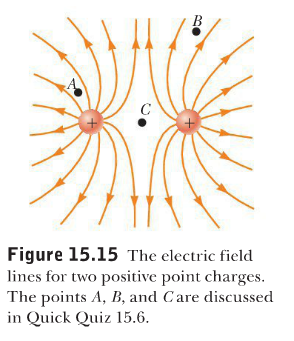Rank the magnitudes of the field at points, A,B and C in figure 15.15 with the largest magnitude first.

a) A,B,C

b) A,C,B

c)C,A,B

d) The answer can't be determined by visual inspection.

A

16

Calculate the magnitude of the flux of a constant electric field of 5.00 N/C in the z-direction through a rectangle with area 4.00m^2 in the xy-plane.

a)0

b)10,0 Nm^2/C

c) 20.0 Nm^2/C

C

17

Calculate the magnitude of the flux of a constant electric field of 5.00 N/C in the z-direction through a rectangle with area 4.00m^2 in the xy-plane.Suppose the electric field of Quick Quiz 15.7 is titled 60 degrees away from the positive z-direction. Calculate the magnitude of the flux through the same area.

a)0

b)10,0 Nm^2/C

c) 20.0 Nm^2/C

B

18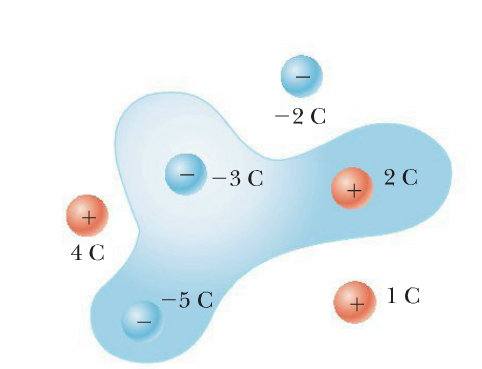Find the electric flux through the surface in the figure. Assume all charges in the shaded area are inside the surface.

a)-(3C)/e0

b)(3C)/e0

c)0

d)(-6C)/e0

D

19

For a closed surface through which the net flux is zero, each of the following four statements could be true. Which of the statements must be true?

a)There are no charges inside the surface

b) The net charge inside the surface is zero.

c) The electric field is zero everywhere on the surface

d) The number of elctric field lines enter the surface equals the number leaving the surface.

B and D

20

If an electron is released from rest in a uniform electric field, does the electric potential energy of the charge-field system.

a) increase

b) decrease

c)remain the same

B

21

If a negatively charged particle is placed at rest in an electric potential field that increases in the positive x-direction, will the particle

a) accelerate in the positive x-direction

b)accelerate in the negative x-direction

c)remain at rest

A

22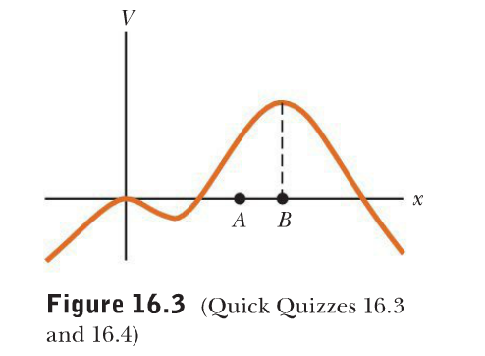Figure is a graph of an electric potential as a function of position. If a positively charged particles is placed at point A, what will its subsequent motion be? Will it

a) go the right

b) go to the left

c)remain at point A

d) oscillate around point B

B

23If a negatively charged particle is placed at point B in Figure 16.3 and given a very small kick to the right, what will its subsequent motion be?

a) go to the right and not return

b)go to the left

c)remain at point B

d)oscillate around point B

D

24

Consider a collection of charges in a give region and suppose all other charges are distant and have a negligible effect. Further, the electric potential is taken to be zero at infinity. If the electric potential at a given pint in the region is zero, which of the following statements must be true?

a) The electric field is zero at that point

b)The electric potential energy is a minimum at that point

c)There is no net charge in the region

d)Some charges in the region are positive, and some are negative

e)The charges have the same sign and are symmetrically arranged around the given point

D

25

A spherical balloon contains a positively charged particle at its center. As the balloon is inflated to a larger volume while the charged particle remains at the center, which of the following are true?

a)The electric potential at the surface the balloon increases

b)The magnitude of the electric field at the surface of the balloon increases

c)The electric flux through the balloon remains the same

d)None of these

C

26

A capacitor is designed so the on e plate is large and the other is small. If the plates are connected to a battery.

a) the large plate has a greater charge than the small plate

b)the large plate has less charge than the small plate

c)the plates have equal,but opposite,charge

C

27

A parallel-plate capacitor is disconnected from a battery, and the plates are pulled a small distance farther apart. Do the following quantities increase, decrease, or stay the same? C

a)increase

b)decrease

c)stay the same

B

28

A parallel-plate capacitor is disconnected from a battery, and the plates are pulled a small distance farther apart. Do the following quantities increase, decrease, or stay the same? Q

a)increase

b)decrease

c)stay the same

C

29

A parallel-plate capacitor is disconnected from a battery, and the plates are pulled a small distance farther apart. Do the following quantities increase, decrease, or stay the same? E between the plates

a)increase

b)decrease

c)stay the same

C

30

A parallel-plate capacitor is disconnected from a battery, and the plates are pulled a small distance farther apart. Do the following quantities increase, decrease, or stay the same?Delta V

a)increase

b)decrease

c)stay the same

A

31

A parallel-plate capacitor is disconnected from a battery, and the plates are pulled a small distance farther apart. Do the following quantities increase, decrease, or stay the same? energy stored in the capacitor

a)increase

b)decrease

c)stay the same

A

32

A fully charged parallel-plate capacitor remains connected to a battery while a dielectric is slid between the plates. Do the following quantities increase, decrease, or stay the same? C

a)increase

b)decrease

c)stay the same

A

33

A fully charged parallel-plate capacitor remains connected to a battery while a dielectric is slid between the plates. Do the following quantities increase, decrease, or stay the same? Q

a)increase

b)decrease

c)stay the same

A

34

A fully charged parallel-plate capacitor remains connected to a battery while a dielectric is slid between the plates. Do the following quantities increase, decrease, or stay the same? E

a)increase

b)decrease

c)stay the same

C

35

A fully charged parallel-plate capacitor remains connected to a battery while a dielectric is slid between the plates. Do the following quantities increase, decrease, or stay the same? delta V

a)increase

b)decrease

c)stay the same

C

36

A fully charged parallel-plate capacitor remains connected to a battery while a dielectric is slid between the plates. Do the following quantities increase, decrease, or stay the same? energy stored in the capacitor

a)increase

b)decrease

c)stay the same

A

37

Consider a parallel-plate capacitor with a dielectric material between the plates. If the temperature of the dielectric increases, does the capacitance

a)decrease

b)increase

c)remain the same

A

38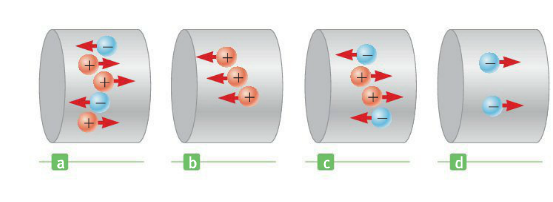Consider Positive and negative charges all moving horizontally with the same speed through the four region in figure 17.2. Rank the magnitudes of the currents in these four regions from lowest to highest.

a) Id ,Ia , Ic ,Ib

b)Ia , Ic ,Ib Id

c)Ic Ia Id Ib

d)Id ,Ib Ic Ia ,

D

39

Suppose a current-carrying wire has a cross-sectional area that gradually becomes smaller along the wire so that the wire has the shape of a very long, truncated cone. How does the drift speed vary along the wire?

a) It slows down as the cross section becomes smaller

b) It speeds up as the cross section becomes smaller

c) It doesn't change

B

40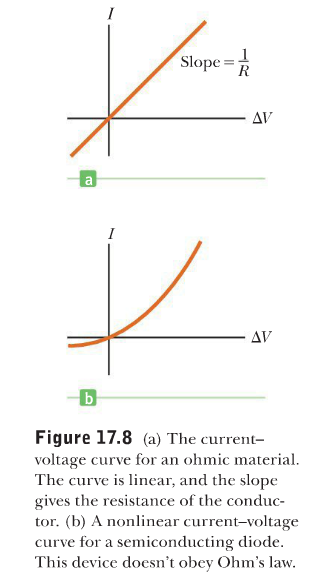In Figure 17.8b does the resistance of the diode>

a) increase

b)decrease as the positive voltage Delta V increase.

B

41

All electric devices are required to have identifying plate that specify their electrical characteristics. The plate on a certain steam iron states that the iron carries current of 6.0 A when connected to a source of 1.20x102 V. What is the resistance of the steam iron?

a) 0.050 ohms

b) 20.0 ohms

c) 36.0 ohms

B

42

Suppose an electrical wire is replaced with one having every linear dimension doubled (i.e. , the length and radius have twice their original values). Does the wire now have.

a) more resistance than before

b ) less resistance

c) the same resistance

B

43

A voltage delta V is applied across the end of a Nichrome heater wire having a cross-sectional A and length L. the same voltage is applied across the ends of a second Nichrome heater wire having a cross-sectional area A and length 2L. Which wire gets hotter?

a)the shorter wire

b)the longer wire

A

44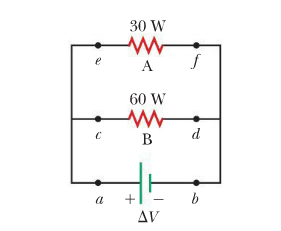For the two resistors show rank the currents at point a through f from largest to smallest.

a)Ia=Ib>Ie=If>Ic=Id

b)Ia=Ib>Ic=Id>Ie=If

c)Ie=If>Ic=Id>Ia=Ib

B

45

Two resistors, A and B, are connected in a series circuit with a battery. The resistance of A is twice that of B. Which resistor dissipates more power?

a)A resistor does

b)B resistor does

A

46

The diameter of wire A is greater than the diameter of wire B, but their lengths and resistivities are identical. For a given voltage difference across the ends, what is the relationship between P A and P B, the dissipated power for wires A and B, respectively?

a)PA =P B

b)P A< PB

c) P A > B

C

47

While discharging the terminal voltage of a battery can never be greater than the emf of the battery

a)true

b) false

A

48

Why does the battery get warm while in use

Because the batteries internal resistance power, is delivered to the battery material, raising its temperature.

49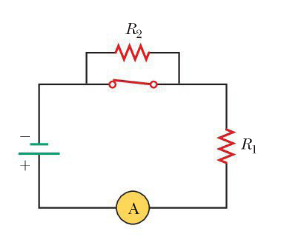The circuit in 18.4 consists of two resistors, a switch, an meter, and a battery. When the switch is closed, power Pc is delivered to the resistor R1. when the switch is open, which of the following statements is true about the power P0 delivered to R1?
(a) P0<Pc
(b) P0=Pc
(c) Po>Pc

A

50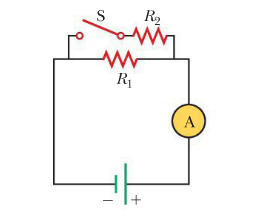n figure 18.7, the current is measured with the ammeter on the right side of the circuit diagram. When the switch is closed, does the reading on the ammeter
(a) increase
(b) decrease
(c) remain the same

A

51

18.7, power P0 is delivered to the resistor R1. When the switch is closed, which of the following is true about the Power Pc delivered to R1? (Neglect the internal resistance of the battery)
(a) Pc<Po
(b) Pc=Po
(c) Pc>Po

B

52

Suppose you have three identical lightbulbs, some wire, and a battery. You connect one lightbulb to the battery and take note of its brightness. You add a second lightbulb, connecting it in parallel with the previous lightbulb, and again take note of the brightness. Repeat the process with the third lightbulb, connecting it in parallel with the other two. As the lightbulbs are added, what happens to
(a) the brightness of the lightbulbs?
(b) the individual currents in the lightbulbs?
(c) the power delivered to the battery?
(d) the lifetime of the battery?

(a) the brightness of the lightbulbs? unchanged
(b) the individual currents in the lightbulbs? unchanged
(c) the power delivered to the battery? increase
(d) the lifetime of the battery? decrease

53

lightbulbs, some wire, and a battery. You connect one lightbulb to the battery and take note of its brightness. You add a second lightbulb, connecting it in series with the previous lightbulb, and again take not of the brightness. Repeat the process with the third lightbulb, connecting it in series with the other two. As the lightbulbs are added, what happens to
(a) the brightness of the lightbulbs?
(b) the individual currents in the lightbulbs?
(c) the power delivered to the battery?
(d) the lifetime of the battery?

(a) the brightness of the lightbulbs? decrease
(b) the individual currents in the lightbulbs? unchanged
(c) the power delivered to the battery? increase
(d) the lifetime of the battery? decrease

54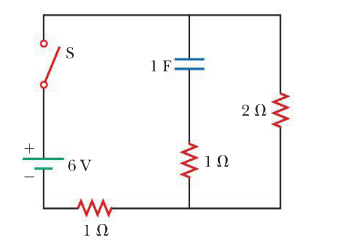The switch in the closed in the figure. After a long time compared with the time constant of the circuit, what will the current be in the 2 Ohm resistor?

a)4A

b)3 A

c) 2A

d) 1A

C

55

A charged particle moves in a straight line through a region of space. Which of the following answers must be true? (Assume any other fields are negligible) The magnetic field
(a) has a magnitude of zero
(b) has a zero component perpendicular to the particles velocity
(c) has a zero component parallel to the particles velocity in that region

B

56

The north pole end of a bar magnet is held near a stationary postively charged piece of plastic. Is the plastic
(a) attracted
(b) repelled
(c) unaffected by the magnet

C

57

A square and a circular loop with the same area lie in the xy plane, where there is a uniform magnetic field (B) pointing at some angle (theta) with respect to the positive Z direction. Each loop carries the same current, int he same direction. Which magnetic torque is larger?
(a) the torque on the square loop
(b) the torque on the circular loop
(c) the torques are the same

C

58

As a charged particle movers freely in a circular path in the presence of a constant magnetic field applied perpendicular to the particles velocity, the particle's kinetic energy
(a) remains constant
(b) increases
(c) decreases

A

59

Which of the following actions would double the magnitude of the magnetic force per unit length between two parallel current-carrying wires? Chose all correct answers
(a) double one of the currents
(b) double the distance between them
(c) reduce the distance between them by half
(d) double both currents

A,C

60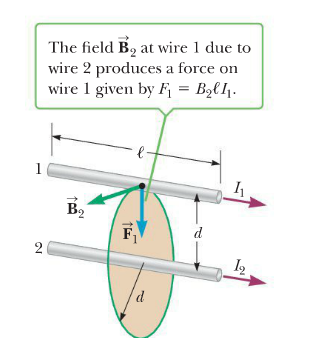In figure 19.28, I1-2A and I2=6a, which of the following is true? Note that F2 represents the magnet of the force on wire 2
(a) F1=3F
(b) F1=F2
(c) F1=F2/3

B